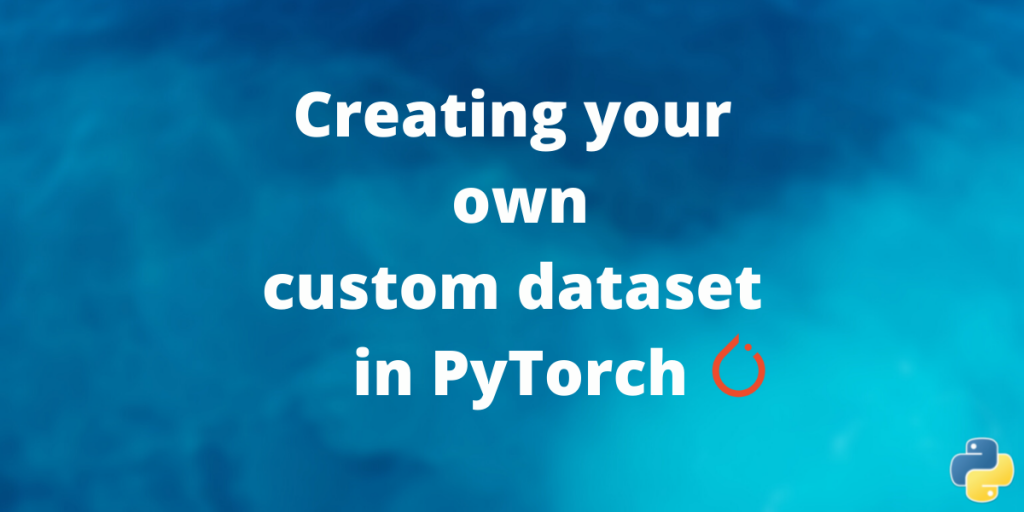# Creating Custom Datasets in PyTorchIn this article, we’ll learn to create a custom dataset for PyTorch.

In machine learning the model the model the as good as the data it is trained upon.

There are many pre-built and standard datasets like the MNIST, CIFAR, and ImageNet which are used for teaching beginners or benchmarking purposes. But there are not many of these pre-defined datasets and if you are working on a relatively new problem, you might not get a pre-defined dataset and you need to train using your own dataset.

In this tutorial we will be understanding some beginner level dataset ceration from custom data using PyTorch.

## Understanding the PyTorch Dataset and DataLoader Classes

Code for processing data samples can get messy and hard to maintain; we ideally want our dataset code to be decoupled from our model training code for better readability and modularity.

PyTorch provides two data primitives: `torch.utils.data.DataLoader `and `torch.utils.data.Dataset` that allow you to use pre-loaded datasets as well as your own data. `Dataset` stores the samples and their corresponding labels, and `DataLoader` wraps an iterable around the `Dataset` to enable easy access to the samples.

So Dataset is the class that is responsible for loading the data from your disk to a computer-readable form. It uses a lazy way to loading memory – It loads memory only when the DataLoader or the user requires to load the data from disk to memory. This is memory efficient because all the images are not stored in the memory at once but read as required.

The torch Dataset class is an abstract class representing the dataset. For creating a custom dataset we can inherit from this Abstract Class. But make sure to define the two very critical functions:

• `__len__` so that `len(dataset)` returns the size of the dataset.
• `__getitem__` to support the indexing such that `dataset[i]` can be used to get iith sample.

The DataLoader simply calls these methods to load the memory. In this article, we will be focusing solely on custom Dataset creation. DataLoaders can be also be extended to a huge extent but it is beyond the scope of this article.

Now that we have learned the basic functioning of `DataLoader`s and `Dataset`s we will be looking at some examples of how it is done in real life.

This is a relatively simple example to load all the images in a folder into a dataset for GAN training. All data are from the same classes so you don’t need to care about labeling for now.

### 1. Initializing the Custom Dataset class

```# Imports
import os
from PIL import Image
from torch.utils.data import Dataset
from natsort import natsorted
from torchvision import datasets, transforms

def __init__(self, main_dir, transform):

self.main_dir = main_dir
self.transform = transform

# List all images in folder and count them
all_imgs = os.listdir(main_dir)
self.total_imgs = natsorted(all_imgs)

```

Now we need to define the two specialized function for our custom dataset.

### 2. Defining __len__ function

This function will allow us to identify the number of items that have been successfully loaded from our custom dataset.

```    def __len__(self):
# Return the previously computed number of images
return len(self.total_imgs)
```

### 3. Defining __getitem__ function

```    def __getitem__(self, idx):
img_loc = os.path.join(self.main_dir, self.total_imgs[idx])

image = Image.open(img_loc).convert("RGB")
# Apply the transformations
tensor_image = self.transform(image)
return tensor_image
```

After you have defined the dataset you can create your own instance using,

```dataset = LoadFromFolder(main_dir="./data", transform=transform)
print(next(iter(dataloader)).shape)  # prints shape of image with single batch
```

Let us say we have a little more complicated problem like cat and dog classifier. We now have to label the images of the dataset. For this, we have a very special PyTorch Dataset Class ImageFolder

Suppose we have the following directory structure:

All the images of cats are in folder cat and all the images of dogs are in folder dogs. If you happen to have the following directory strucutre you create your dataset using

```from torchvision.datasets import ImageFolder
dataset = ImageFolder(root="./root", transform=transform)
print(next(iter(dataloader)).shape)  # prints shape of image with single batch
```

You can always alter how the images are labelled and loaded by inherting from ImageFolder class.

If you are working with audio, the same techniques are applicable in the case of audio too. The only thing that changes is the way the length of the dataset is measured and files are loaded in memory.

```from torch.utils.data import Dataset

class SpectrogramDataset(Dataset):

def __init__(self,file_label_ds,  transform, audio_path=""):
self.ds= file_label_ds
self.transform = transform
self.audio_path=audio_path

# The length of the dataset
def __len__(self):
return len(self.ds)

# Load of item in folder
def __getitem__(self, index):
file,label=self.ds[index]
x=self.transform(self.audio_path+file)
return x, file, label

```
```# file_label_ds is a dataset that gives you the file name and label.
dataset = SpectrogramDataset(file_label_ds, transform)
```

## Conclusion

This brings us to the end of the article. Stay tuned for more articles on Deep Learning and PyTorch.